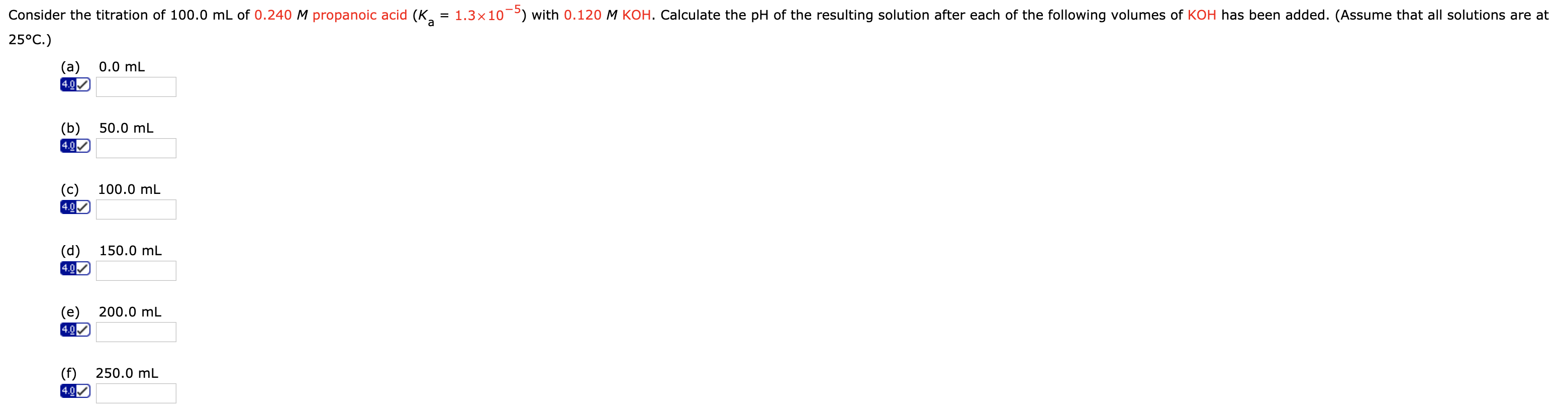# Consider the titration of 100.0 mL of 0.240 M propanoic acid (Ka = 1.3 ✕ 10−5) with 0.120 M KOH. Calculate the pH of the resulting solution after each of the following volumes of KOH has been added. (Assume that all solutions are at 25°C.) (f) 250 ml